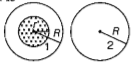Two conducting circular loops of radii R1 and R2 are placed in the same plane with their centres coinciding. If R1 >> R2, the mutual inductance M between them will be directly proportional to

(1) R1/R2

(2) R2/R1

(3) ${R}_{1}^{2}/{R}_{2}$

(4) ${R}_{2}^{2}/{R}_{1}$

Subtopic:  Mutual Inductance |
To view explanation, please take trial in the course below.
NEET 2022 - Target Batch - Aryan Raj Singh
To view explanation, please take trial in the course below.
NEET 2022 - Target Batch - Aryan Raj Singh

Difficulty Level:

Two conducting circular loops of radii  are placed in the same plane with their centres coinciding. If ${\mathrm{R}}_{1}>>{\mathrm{R}}_{2}$, the mutual inductance M between them will be directly proportional to:

1. $\frac{{\mathrm{R}}_{1}^{2}}{{\mathrm{R}}_{2}}$

2. $\frac{{\mathrm{R}}_{2}^{2}}{{\mathrm{R}}_{1}}$

3. $\frac{{\mathrm{R}}_{1}}{{\mathrm{R}}_{2}}$

4. $\frac{{\mathrm{R}}_{2}}{{\mathrm{R}}_{1}}$

Subtopic:  Mutual Inductance |

Difficulty Level:

The magnetic flux linked with a coil (in Wb) is given by the equation $\mathrm{\varphi }=5{\mathrm{t}}^{2}+3\mathrm{t}+60$
The magnitude of induced emf in the coil at the fourth second will be:

1.  33 V

2.  43 V

3.  108 V

4.  10 V

Subtopic:  Faraday's Law & Lenz Law |

Difficulty Level:

A wheel with 20 metallic spokes, each 1 m long, is rotated with a speed of 120 rpm in a plane perpendicular to a magnetic field of 0.4 G. The induced emf between the axle and rim of the wheel will be,

1.

2.

3.

4.  2.51 V

Subtopic:  Motional emf |

Difficulty Level:

In which of the following devices, the eddy current effect is not used?

1. Electric heater

2. Induction furnace

3. Magnetic braking in train

4. Electromagnet

Subtopic:  Eddy Current |

Difficulty Level:

A 800 turn coil of effective area 0.05 ${m}^{2}$ is kept perpendicular to a magnetic field . When the plane of the coil is rotated by 90$°$ around any of its coplanar axis in 0.1 s, the emf induced in the coil will be:

1. 0.02 V

2. 2 V

3. 0.2 V

4.

Subtopic:  Faraday's Law & Lenz Law |

Difficulty Level:

A cycle wheel of radius 0.5 m is rotated with a constant angular velocity of 10 rad/s in a region of a magnetic field of 0.1 T which is perpendicular to the plane of the wheel. The EMF generated between its centre and the rim is:

1. 0.25 V

2. 0.125 V

3. 0.5 V

4. zero

Subtopic:  Motional emf |

Difficulty Level:

The magnetic potential energy stored in a certain inductor is 25 mJ, when the current in the inductor is 60 mA. This inductor is of inductance :

1. 0.138 H

2. 138.88 H

3. 1.389 H

4. 13.89 H

Subtopic:  Self - Inductance |

Difficulty Level:

A long solenoid of diameter 0.1m has 2$×{10}^{4}$ turns per meter. At the centre of the solenoid, a coil of 100 turns and radius 0.01m is placed with its axis coinciding with the solenoid's axis.  The current in the solenoid reduces at a constant rate to 0 A from 4A in 0.05s. If the resistance of the coil is $10{\pi }^{2}\Omega$, the total charge flowing through the coil during this time is

1. 32$\mathrm{\pi \mu C}$

2. 16$\mu C$

3. 32$\mu C$

4. 16$\mathrm{\pi \mu C}$

Subtopic:  Faraday's Law & Lenz Law |

Difficulty Level:

A uniform magnetic field is restricted within a region of radius r. The magnetic field changes with time at a rate $\frac{dB}{dt}$. Loop 2 of radius R is outside the region of magnetic field as shown in the figure. Then the emf generated is-(1) zero in loop 1 and zero in loop 2

(2)

(3)

(4)

Subtopic:  Faraday's Law & Lenz Law |
To view explanation, please take trial in the course below.
NEET 2022 - Target Batch - Aryan Raj Singh
To view explanation, please take trial in the course below.
NEET 2022 - Target Batch - Aryan Raj Singh

Difficulty Level: